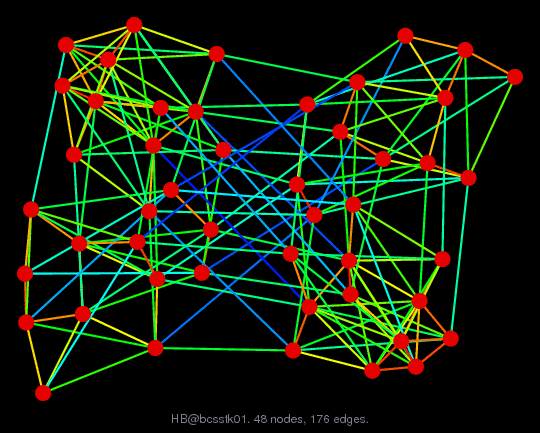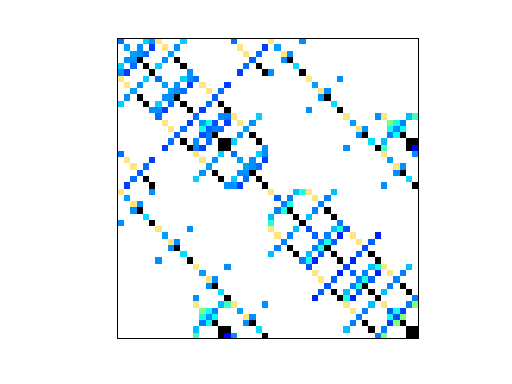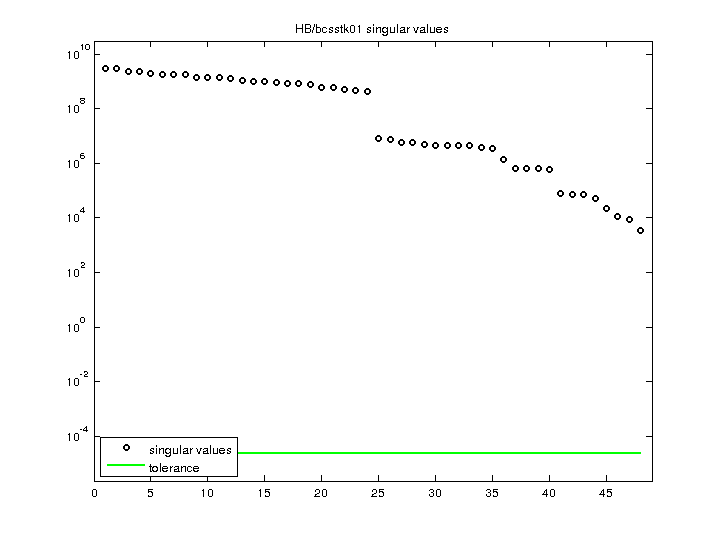Matrix: HB/bcsstk01

Description: SYMMETRIC STIFFNESS MATRIX SMALL GENERALIZED EIGENVALUE PROBLEM(undirected graph drawing)• Matrix group: HB
• download as a MATLAB mat-file, file size: 2 KB. Use UFget(23) or UFget('HB/bcsstk01') in MATLAB.

 Matrix properties number of rows 48 number of columns 48 nonzeros 400 structural full rank? yes structural rank 48 # of blocks from dmperm 1 # strongly connected comp. 1 explicit zero entries 0 nonzero pattern symmetry symmetric numeric value symmetry symmetric type real structure symmetric Cholesky candidate? yes positive definite? yes

 author J. Lewis editor I. Duff, R. Grimes, J. Lewis date 1982 kind structural problem 2D/3D problem? yes

 Ordering statistics: result nnz(chol(P*(A+A'+s*I)*P')) with AMD 489 Cholesky flop count 6.0e+03 nnz(L+U), no partial pivoting, with AMD 930 nnz(V) for QR, upper bound nnz(L) for LU, with COLAMD 651 nnz(R) for QR, upper bound nnz(U) for LU, with COLAMD 944

 SVD-based statistics: norm(A) 3.01518e+09 min(svd(A)) 3417.27 cond(A) 882336 rank(A) 48 sprank(A)-rank(A) 0 null space dimension 0 full numerical rank? yes

 singular values (MAT file): click here SVD method used: s = svd (full (A)) status: ok